C2000 Solar MPPT tutorial Pt/2

In this second part of the C2000 Solar MPPT Tutorial, the hardware and circuit design will be looked at in greater depth.  The schematic for the system is posted again below for reference, which can be enlarged by simply clicking on it.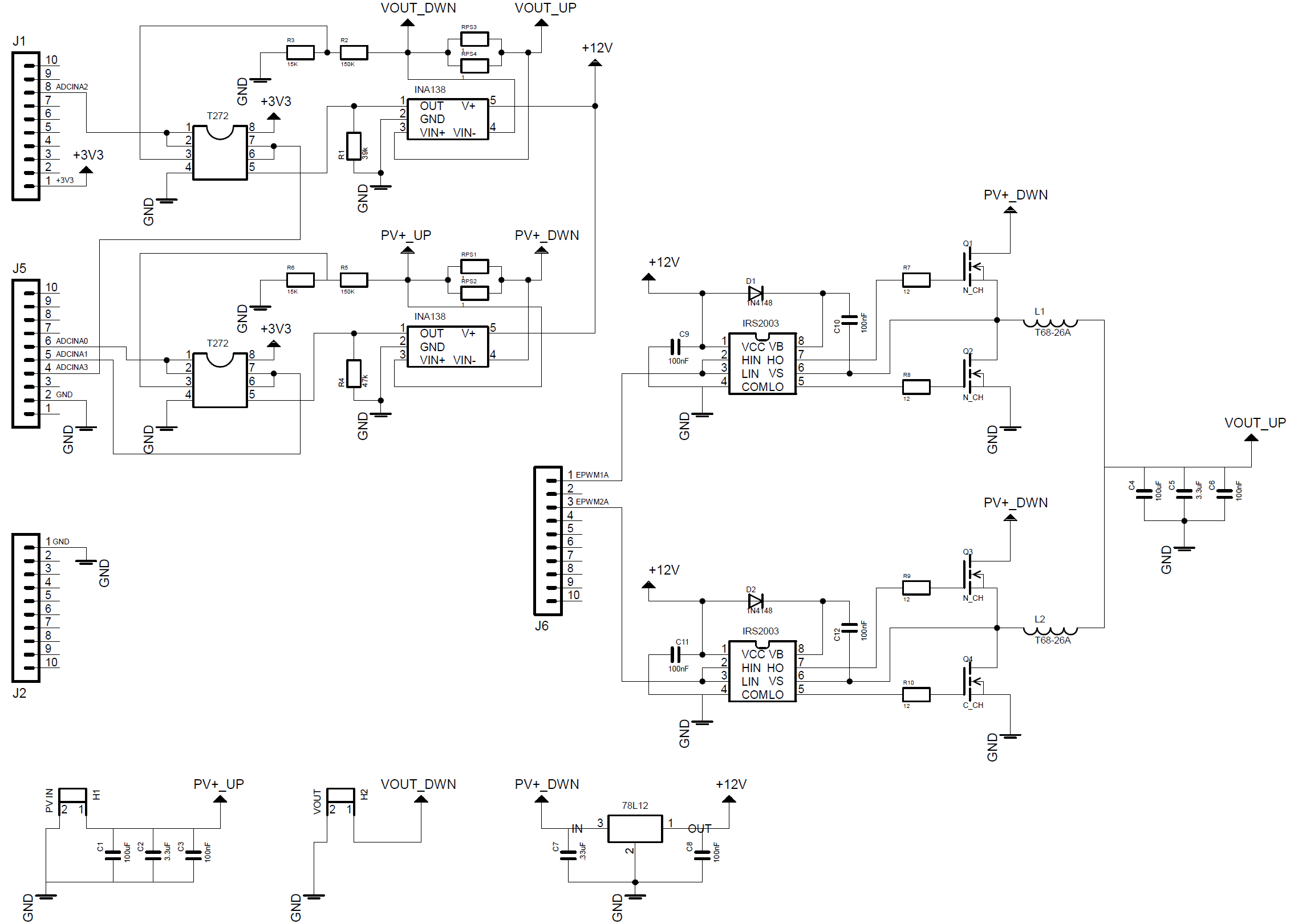Buck Converter Design

The first step was to design the buck circuit, this is determined by the output parameters of the system and it’s load.  For the first prototype based around the panel purchased for testing, it was decided to aim for a 12V output, therefore a maximum current of 750mA (assuming 10% losses).

When calculating a buck circuit the frequency of operation, inductor size and output capacitor size are important, as they determine the current and voltage ripple size.  It is desirable to have as smaller current and voltage ripple as possible.  A large current ripple can cause additional losses in a system, as there maybe times when the peak current is greater than the load requirements.  A large voltage ripple is obviously not desirable, good quality regulated power supplies have very low voltage ripples.

A general rule is the higher the frequency the smaller the inductor and output capacitor size, and a smaller inductor and capacitor size generally lowers the system cost.  However higher PWM frequencies decrease the system efficiency due to switching losses in the mosfets, so a trade off has to be reached which meets the design constraints of the end system.

For this system a PWM frequency of 15kHz was chosen, based on this, the solar panel and other design parameters for the buck circuit calculations can be performed.  First we can determine the system duty cycle at MPP, note the duty cycle will change to track the MPP with differing irradiance.  A figure of 90% was used for the buck converter efficiency,a typically buck converter efficiency is 90% or greater.Next an ideal current ripple can be determined, it is important to note that the below formulae used only determines a single phase current ripple.ΔIL is the inductor ripple current, and in this case a 30% figure was used for the multiplier. Now that the current ripple is know the inductor size can be calculated with the following equation.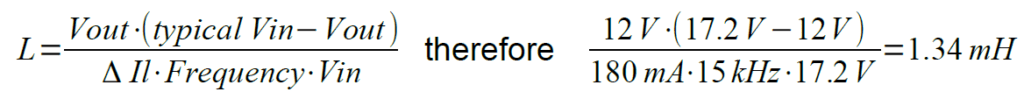Using two 1.34mH inductors on each phase will ensure the inductor ripple current is effectively halved, knowing this an inductor ripple current of 90mA can be used to calculate the minimum output capacitor size.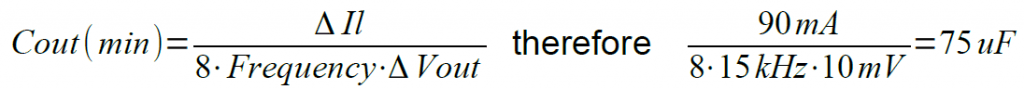Δvout is the desired ripple voltage.  The constant 8 is determined by the simplification of an equation, which can be found in various sources, one such source is an Application Note by On-Semiconductor AND9135/D.

There are two other factors for the inductor and capacitor that are important to consider: the inductor peak current and the capacitors Equivalent Series Resistance (ESR).  The inductor for this project will be hand wound using a toroidal core, which will be covered shortly.  The capacitors ESR can affect the reliability of the capacitor.  A capacitor will dissipate power as heat depending on it’s ESR, so a low ESR is desirable as excessive heating will shorten the life of a capacitor and be less efficient.  For this early prototype, cheap off the shelf capacitors were used as their reliability over time was not a concern at this stage.

The inductors were constructed using a T68-26A toroidal core, this core has a nominal inductance or Al value of 58nH.  The following equation was used to determine how many turns of wire the core would need.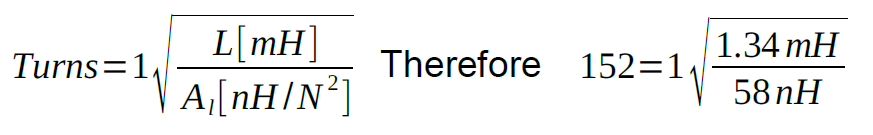A 0.3mm outside diameter enamelled cable was chosen, this has a maximum current rating of 1.4A.  Then a very useful website found here was used, this allows you to calculate the total length of cable required based on the toroidal core dimensions and cable diameter. Using a vice the cores were both wound and then measured using a LCR meter and measured at 1.3mH, an image below shows the hand winding process used.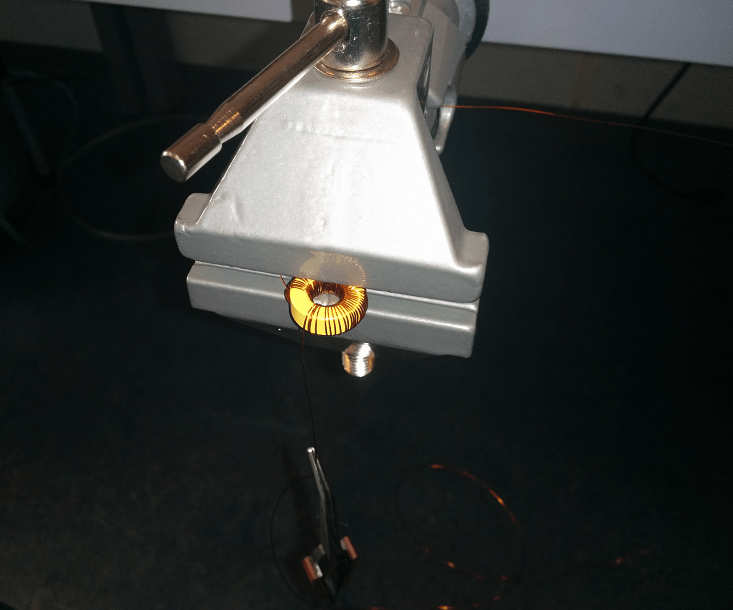MOSFET Losses

For the prototype system Vishay IRFI640G MOSFETs were used, these are not the most efficient having a high RDSON value (180mΩ), but they were stocked at the time of writing.

The power losses from the High Side and Low Side MOSFETs are a combination of conduction and AC switching losses.  The conduction losses are a result of I²R losses inside the MOSFET when it is fully on, and the switching losses are the result of the MOSFET transitions from its on and off states.  Some example calculations will now be shown using data from the IRFI640G datasheet and various sources, on a synchronous MOSFET buck circuit efficiency.The first equation is for the High Side MOSFET and is based on the Vmp of the solar panel at maximum output current running through each interleaved phase.The next equation is for the Low Side MOSFET using the same current figure.The results from these two equations can be combined to work out the overall efficiency, the losses will also be multiplied by a factor of 2 as this is for a two phase interleaved circuit.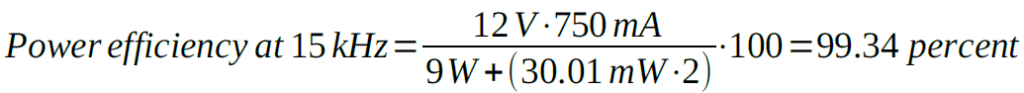Now if we increase the PWM frequency to 150kHz, the circuit losses will also be shown to increase.The switching losses in this next example will be shown to increase with increasing current, the next example uses a 15kHz switching frequency but with 2A, increasing the overall power to 24W.A MOSFET with a lower RDS(ON) will have lower conduction losses, but it will typically have a higher QG (Gate Charge) resulting in higher switching losses.  Therefore a careful balance between these characteristics should be found to maximise the circuits efficiency.  Taking into account the nominal parameters of the system, such as input voltage, output current, switching frequency and duty cycle, will allow the best efficiency to be achieved.  This will often involve using MOSFETs with different characteristics for the High and Low Side drivers.

MOSFET Driver Circuit

The Half-Bridge (H-Bridge) driver is an IC designed specifically for driving MOSFETs. The IC takes the incoming PWM signal, and then drives two outputs for a High and a Low Side MOSFET.  This type of H-bridge is often used to to drive motors, but has other applications such as the following example.  The IC used is a IRS2003 half bridge driver made by International Rectifier, the image below shows an example circuit from the datasheet.The IRS2003 allows 2 PWM signals to be connected to HIN and LINNot this gives the user the opportunity to fine tune the dead-band switching of the MOSFETs.  The capacitor wired between VB and VS along with the diode form a charge pump, this allows the drive voltage to the MOSFETs to be almost doubled.  HIN and LINNot in this case are wired together and supplied with the same PWM signal, the IRS2003 has internal timing to ensure the High Side MOSFET, and the Low Side MOSFET are never on at the same time.  The capacitor between VB and VS needs to be sized to ensure it can drive enough current to the gate of the chosen MOSFET, over coming the gate capacitance.

There are four ADC ports used on this project, two sampling voltage and two sampling current.  The hardware employed to sample the voltage signals will be covered first, followed by the current sampling circuit.  But first a brief introduction to the TMS320F28027 ADC.

The ADC measures voltage from 0V to 3.3V, with a 12bit resolution.  It is important to not exceed the input voltage of the microcontrollers GPIO pins, the TMS320F28027 has a maximum input voltage of 3.63V.  Using this information the step resolution for the ADC can be calculated.The input on the ADC also has a small internal capacitance and resistance, this is used for Sample and Hold acquisition depending on the characteristics of the circuit being sampled. The internal ADC circuit taken from the TMS320x2802x datasheet is shown below.To ensure the readings being sampled are as accurate as possible, the source resistance or RS shown in the above image ideally needs to be as small as possible.  This will be achieved by placing an opamp configured as a unity gain buffer in all the ADC sample circuits.  The unity gain buffer will ensure a high input impedance, therefore reducing loading effects on the sampled circuitry to a minimum, as well as offering a very low output impedance to the C2000 internal ADC circuit.  Rail to rail opamps were used and supplied with 3.3V, this ensured the voltage passed to the ADC would not exceed this, thus ensuring the system has ADC protection built in.

The voltage sampling circuits consist of a simple potential divider, the maximum voltage the solar panel can produce is 21.6V when open circuit.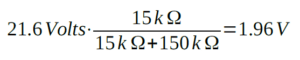The same resistor and opamp configuration is used for the input and output voltage measurement.  A more complex opamp circuit could have been used with offset, to fully exploit the range of the ADC, however this would provide more than enough accuracy for the prototype.

The current sampling circuit involved a slightly more complex approach.  The circuit would revolve around a Texas Instruments INA138 High Side Measurement current shunt monitor. The INA138 is basically a differential amplifier housed inside a small package, with a wide operating voltage.  The INA138 would be supplied with 12V, this then allows for a greater range to be measured around the 0V-3.3V range and was also the second supply voltage available for this circuit.  A typical configuration taken from the datasheet is shown in the image below.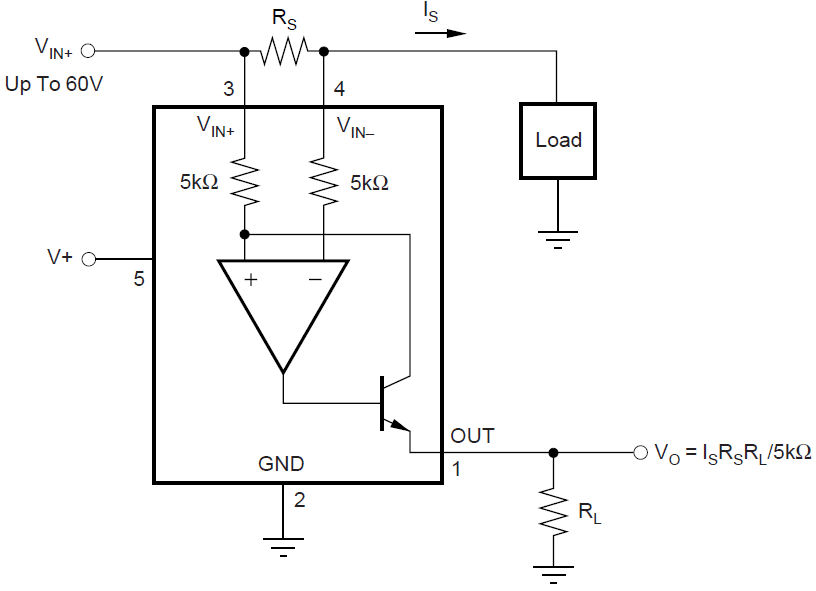There are differences in input and output current so two formulas would be needed to ensure the range was correct.  The shunt resistors comprised of two 1Ω (1%) resistors in parallel, so the combined value becomes 0.5Ω.  The parallel resistors were measured and the actual value was approximately 0.47Ω.  A quick calculation to check these could withstand the power loads was made.This was not the best long term solution, but within tolerance for the 1% resistors in parallel.

The shunt resistor is connected directly across the internal differential amplifiers inputs. The calculations for the INA138 are fairly simple, the gain resistor soldered externally determines the overall gain of the device.  The datasheet for the INA138 states that the device has a gain of 1 with a 5kΩ resistor, gain of 2 with a 10kΩ, gain of 5 with a 25kΩ and so on and so forth. The input and output current gain resistors were calculated as follows.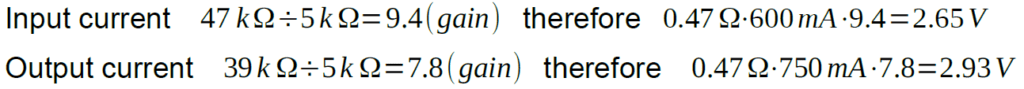These values would ensure the output from both the current feedback circuits falls in-line with the ADC input.

Schematic Design and Layout

Certain aspects of the design were simulated in OrCad 16.6 first, to back-up the theory with simulation.  Then the design was taking over to EagleCad to enable faster prototyping.  Component symbols and foot prints where designed for all the non standard parts, ensuring these were all compatible with the LPKF milling machine used.  Once the schematic design was complete and the Electrical Rule Check (ERC) and Design Rule Check (DRC) were satisfactory, a schematic design was made for a two layer board.  The layout kept the PWM and digital switching side and analogue circuitry away from each other to avoid unnecessary noise.  The below image shows the final PCB prototype layout.The next part of this tutorial will go more in-depth into the C2000 software and Perturb and Observe algorithm, also including a downloadable version of the C code.

I take great care when writing all the tutorials and articles, ensuring all the code is fully tested to avoid issues for my readers.  All this takes time and a great deal of work, so please support the site by using the Adfly links etc.  If you have found this useful or have any problems implementing, please feel free to leave a comment and I will do my best to help.

C2000 Solar MPPT tutorial Pt/1

This series of four posts will cover a project build for a C2000 Solar MPPT Tutorial.  The Maximum Power Point Tracker (MPPT) circuit is based around an interleaved synchronous buck circuit topology.  The microcontroller used is the Texas Instruments (TI) C2000 family, the C2000 Launchpad is used which has a TMS320F28027 chip on-board.  Considerable research was carried out on this project, as there was no prior knowledge of the C2000, all the relevant peripherals were tested separately and then brought together and tweaked for the final code.  TI provides all the documentation required, which can be found here and for this tutorial the ADC, ePWM and the F2802x Peripheral Driver Library Users’ Guide were referenced.

The C2000 solar MPPT tutorial can also be used as a guide if designing a circuit for other microcontrollers such as Arduino, as the electronic principles and some of the code is transferable to any platform.

The image below shows the final prototype MPPT PCB with the C2000 Launchpad secured on top via the header connections.The video below shows the system set-up, allowing the Perturb and Observe algorithm to be tuned.  There is also a second video demonstrating the algorithm tracking the maximum power point of the solar panel, this can be found on my YouTube account and will be embedded in the final tutorial.

In this part a basic overview of the hardware design will be covered, further tutorial parts will cover the following areas in greater detail:

• Buck circuit calculation, a look at MOSFET efficiency, current and voltage sampling and circuit design and layout
• Software approach including phase shifted PWM, ADC sampling and the Perturb and Observe algorithm
• Final set-up, testing and tuning

Maximum Power Point Tracking

Maximum power point tracking is employed, to ensure the maximum power is extracted from a solar panel.  In order to understand this further we first need to look at the power curve characteristics of a solar panel, the image below shows a Suntech STP230-20Wd (230W panel) power curve.The maximum power point (MPP) of a solar panel lies at the knee of the current and voltage curve.  Reading the datasheet on the Suntech panel tells us that Vmp (voltage at maximum power point) is 29.8V and Imp (current at maximum power point) is 7.72A, locating the intersection point of these two values, it can be seen that the MPP is at the knee of the curve. The graph also illustrates that the voltage variation is much less compared to the current only with differing irradiance, however the current varies linearly with solar intensity.  Temperature also affects the power output from a panel, current increases slightly with an increase in temperature, whereas voltage decreases with an increase in temperature.  As the voltage is affected by temperature more than the current, voltage calculations need to be considered when large string arrays are used, to ensure the system meets the requirements of the inverter used.

The panel has an internal resistance which changes dynamically with differing irradiance levels.  So if a static load is connected directly to a panel and its resistance is higher or lower than the panels internal resistance at MPP, then the power drawn from the panel will be less than the maximum available.  Taking a simple example under bright midday sun, say we connected the Suntech STP230-20Wd directly to a 12V lead acid battery, the panel voltage would be dragged down near to the load voltage of the battery as the batteries resistance is lower than the panels.  With a quick calculation the panel is now outputting 12V and 7.72A, therefore 93W, this equates to a loss of 137W or 60%. Obviously this is an extreme example, but even using a 24V battery would still equate to a 20% loss, which is far from efficient.

This is where MPPT comes into play.  MPPT circuits can be based on various switch mode power supply (SMPS) topologies, they generally have a fixed frequency but varying duty cycle.  The duty cycle is controlled via an algorithm so as to track the changing MPP, the output power is determined by the efficiency of the circuit and usually closely matches the incoming power within 3-10% (typical losses).  The output voltage and current will not necessarily be fixed under changing irradiance conditions depending on the system employed, so further circuitry maybe required or a more elaborate algorithm.

System Overview

The image below shows an overview of the final systemThe solar panel in the diagram will be represented in real life by a 10W panel purchased from Ebay, this has a Vmp of 17.2V and an Imp of 580mA.  Four ADC inputs and 2 PWM outputs will be used on the C2000.  The input voltage and current and output voltage and current will both be monitored, so the input and output power can be determined.  A pair of half bridge driver integrated circuits (IC) will be used to drive the four N-Channel MOSFETs. There is also an auxiliary supply, this was used to power a 12V linear regulator which powered the half bridge drivers.  The 12V regulator could also be powered directly from the panel, which was used in testing under bright sun conditions.

The chosen SMPS topology will be based on a synchronous buck converter circuit, there will be two of these in parallel forming an interleaved design.  Using an interleaved approach is over kill for the 10W panel prototype, but it gives the system scalability for future iterations.  The synchronous buck design was chosen as it offers a higher efficiency, these design choices will become clear as the tutorial progresses.

Interleaved Synchronous Buck Converter

The buck converter circuit will be over viewed as this forms a large part of the system design, this will naturally lead into the advantages of the synchronous and interleaved design chosen.  A boost circuit could quite easily be implemented in place of buck design, but in this case the target output voltage was 12V.

A buck converter is basically a small DC to DC converter.  The main principle at work in a buck converter, is the tendency for an inductor to resist changes in current.  A buck converter output voltage will always be lower or the same as the input voltage.  A simplified schematic of a buck converter can be seen in the below image.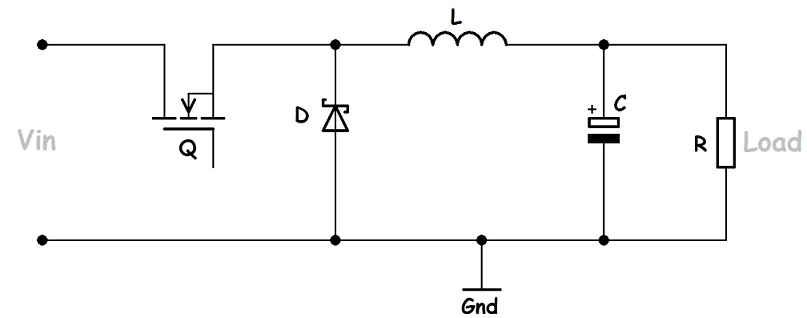A buck converter relies on a switch to change or reduce the current flowing through the inductor, the switch usually takes the form of a MOSFET (Q).

1. When the MOSFETs gate is saturated effectively closing the switch, current flows through the inductor (L) in a clockwise direction into the load (R) and also charging the output capacitor (C).  At this point the voltage on the cathode of the diode is positive, therefore the diode (D) is blocking any flow of current and is said to be reverse biased.  The Instantaneous current flow into the load from Vin is slow, as energy is stored in the inductor as it’s magnetic field increases.  So during the on phase of the MOSFET, energy is loaded into the inductor.
2. When the MOSFET is switched off, the voltage across the inductor is reversed.  The inductors magnetic field begins to collapse, this collapse releases the stored energy allowing current to flow from the inductor into the load.  The diode now has a negative voltage on the cathode so becomes forward biased.  Therefore the inductors discharge current flows in a clockwise direction through the load and back through the diode. Once the inductors energy has fallen below a certain threshold, the load voltage falls and the capacitor becomes the main source of current, ensuring the load is still supplied until the next switching cycle begins.  To ensure Continuous Conduction Mode (CCM) the inductor must not be fully discharged before the MOSFET is switch on again, and the cycle repeats.

Now that the basic concept of a buck converter has been explained the synchronous design can be covered.  The synchronous design simply replaces the diode with a second MOSFET, this eliminates the losses incurred by the forward voltage drop across the diode, thus making the circuit more efficient.  This is slightly more complex to implement, as the second mosfet switching needs to be carefully timed with the switching of the first mosfet.  It is essential to ensure that both are never on at the same time, or the current will have a direct path to ground, effectively causing a short circuit.  The MOSFET switching is effectively 180 degrees out of phase, with a short delay period between each transition referred to as a Dead-Band.  A dead-band is usually a common feature of most half bridge drivers, the C2000 also allows for the dead-band to be programmed in and tuned, so in reality with modern microcontrollers this is quite simple to implement.

The interleaved design simply takes two synchronous buck circuits and places them in parallel, the main components MOSFETs and inductor are individual to each circuit, but they share a common input and output as well as the same input and output capacitors.  Using an interleaved design reduces the current ripple by half, as each interleaved phase shares the total current.  The shared current allows smaller inductors and capacitors to be used, which can also reduce system size and cost.  Additionally this systems PWM frequency was chosen to be 15kHz as it reduces the switching losses in the MOSFETs, but increases the current ripple and also the inductor size.  By using the interleaved approach it then helps to negate these factors.  These trade-offs will be covered in more detail in the hardware section of this tutorial, where MOSFET losses will be covered.

10W Solar Panel

As mentioned previously the solar panel was purchased from Ebay for around £20.  It’s a polycrystalline panel, which is encased in an aluminium frame and comes with 2 large croc clip leads and a small diode box.  The panel seems pretty good quality and appears to be weather proofed, the diode box is not sealed very well but as it sits under the panel I don’t believe there would be any issues.  Have enclosed 2 shots of the panel, and I can probably dig out the links to the company if anyone is interested.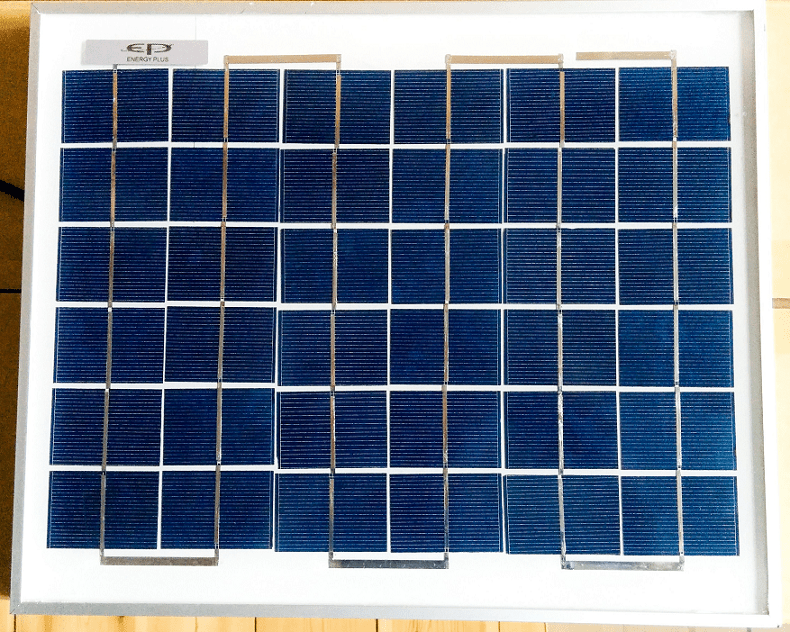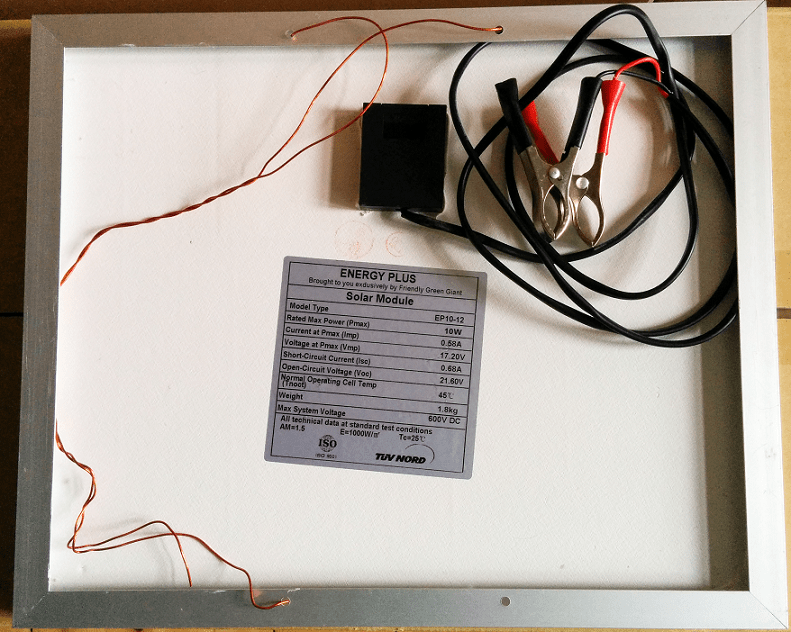System SchematicThe next part of this tutorial will go more in-depth into the circuit calculations and schematic design.

I take great care when writing all the tutorials and articles, ensuring all the code is fully tested to avoid issues for my readers.  All this takes time and a great deal of work, so please support the site by using the Adfly links etc.  If you have found this useful or have any problems implementing, please feel free to leave a comment and I will do my best to help.